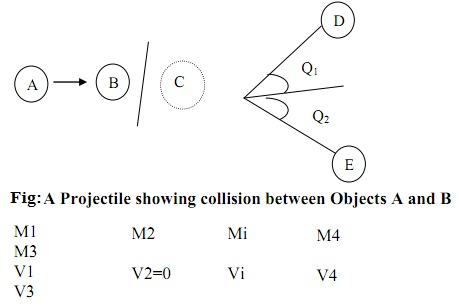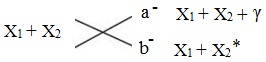#### Energetic of Nuclear Radiation, Chemistry tutorial

Introduction:

Throughout a nuclear reaction between the atomic nucleus and the other atomic particle, three different methods are possible. These are:

a) Nuclear transmutation (in which new nucleus are made)

b) Inelastic scattering (that is, in which original nucleus are applied to a higher energy state)

c) Elastic scattering (that is, in which the nucleus remain uncharged). The mass and energy relationship projectiles interact by a nucleus are brought to fore.

Conservation Laws in Nuclear Interaction:

All the conservation laws are applicable in nuclear reactions, these laws are the:

a) Conservation of total energy ΔE = 0

b) Conservation of the linear momentum ΔP = 0

c) Conservation of net change ΔZ = 0

d) Conservation of mass number ΔA = 0

e) Conservation of spin ΔI = 0

Consider the figure shown below:Fig: Projectile showing collision

This describes a projectile in which substance A collide with target atom B, making intermediate system C. The C system split to product D and E. This is nuclear reaction V1 is > 0 but V2 is made to be zero.

Conservation of Used Energy:

ΔE = ΔE product - ΔE reactant = 0

As the linear momentum is a vector quantity,

P1 + 0 = P3 Cos θ - P4 Cos θ4

P3 Sin θ = P4 Sin θ4

It will be noted that some of the laws earlier stated ((d) and (e)) are not for all time followed in high energy reactions in which latest elementary particles might be found. Supposing that mass of the particle was independent of its velocity the kinetic energy equation as represented by Newton:

Ekin = 1/2 Mv2

The Mass Energy:

For radioactive decay, the energy numeration is represented by its Q value.

Q (MeV) = -931.5 Δmo

Here, Δmo - M3o + Mo4 - Mio - M2o

Whenever mass disappears in the reaction (ΔMo < 0) energy is discharged, then the reaction is stated to be exoergic and 'Q' is positive.

For Q < 0 the reaction is endoergic as ΔMo > 0

Ekin = (m - mo)c2 can be separated to five terms if we state:

Eo mass = Moc2 and EKaf = Mc2

Then Etot = Ekin + Eomass

It is as well noted that: Etot = Ekin + Epot

Therefore: Etot = Ekin + Eomass = Ekin + Epot

Thus; Emass = Epot

Here Ekin is translational, rotational, vibrational energy and Eomass = mass energy, Epot = gravitational, surface energy, electrostatic energy, chemical binding energy and so on.

To have an Etot that comprises atomic masses in their grounds state Eomass, the excitation energy of the nucleus above its ground state Eexc, the absorption or emission of protons in the reaction Ev and c reaction between the charged particles, the electrostatic potential (that is, Coulomb) energy Ecol, and the columb energy should be zero or positive (that is, repulsive). The incoming projective should have adequate kinetic energy to overcome any repulsion. In the procedure of reaction of repulsion of charged particles, product yields in greater kinetic energy.

Etot = 6rm + 6cone - EoMeG + Exec + Er

Elastic scattering:

The elastic scattering energy is replaced between the projectile and the target nucleus however the value of 'Q' is zero. A significant elastic scattering reaction is a nuclear reactor comprises the slowing down of neutrons from kinetic energies, which they have whenever emitted in nuclear fission. The neutrons are slowed down to energies comparable to those of a neutron of gas at the temperature of the material in which they are moving; therefore they are termed as thermal neutrons.

Ekin = KT

Here, Ekin is kinetic energy, 'T' is temperature and 'K' is the reaction constant.

The method of slowing down of energetic neutron to low kinetic energies is termed as moderation.

Inelastic Scattering:

In this group of nuclear reaction termed as inelastic scattering, part of the kinetic energy of the projectile is converted to the target nucleus as excitation energy devoid of changing the values of A or Z of either target or projectile. If the projectile is a heavy ion, it might as well become excited. Though, the collision of the projectile and target nucleus making the product does yield in a value of 'Q' more than zero.Fig: Inelastic Scattering

The reaction path (a) points out that the energy 'Q' is emitted as a γ ray.  In the reaction path (b), the Q is retained as the excitation energy of the target comprise.

In case of the inelastic scattering reaction of the formation of an isomer of Ag by the irradiation of 107Ag by neutrons;

107Ag (n, n'); 107m Ag → 107Ag + γ

As an illustration of nuclear transmutation consider the given:

147N x 42He → (189F)* →1718O + 1H

ΔMo = (m3o + m4o - m1o - m2o)

Δmo = (16.999131 + 1007325 - 14.003074 - 4.002603)

Δmo = 0.001279(u)

The Compound Nucleus Model:

If Eokn > Ecb, the attractive nuclear force dominate and the particles is absorbed via the target nucleus. Supposing Q > 0, the Eomass reduces. This signifies that, Eexe increases and the system is converted to excited compound nucleus.

Therefore, the excitation of the compound nucleus is:

Eexe = Q + EoKin

Tutorsglobe: A way to secure high grade in your curriculum (Online Tutoring)